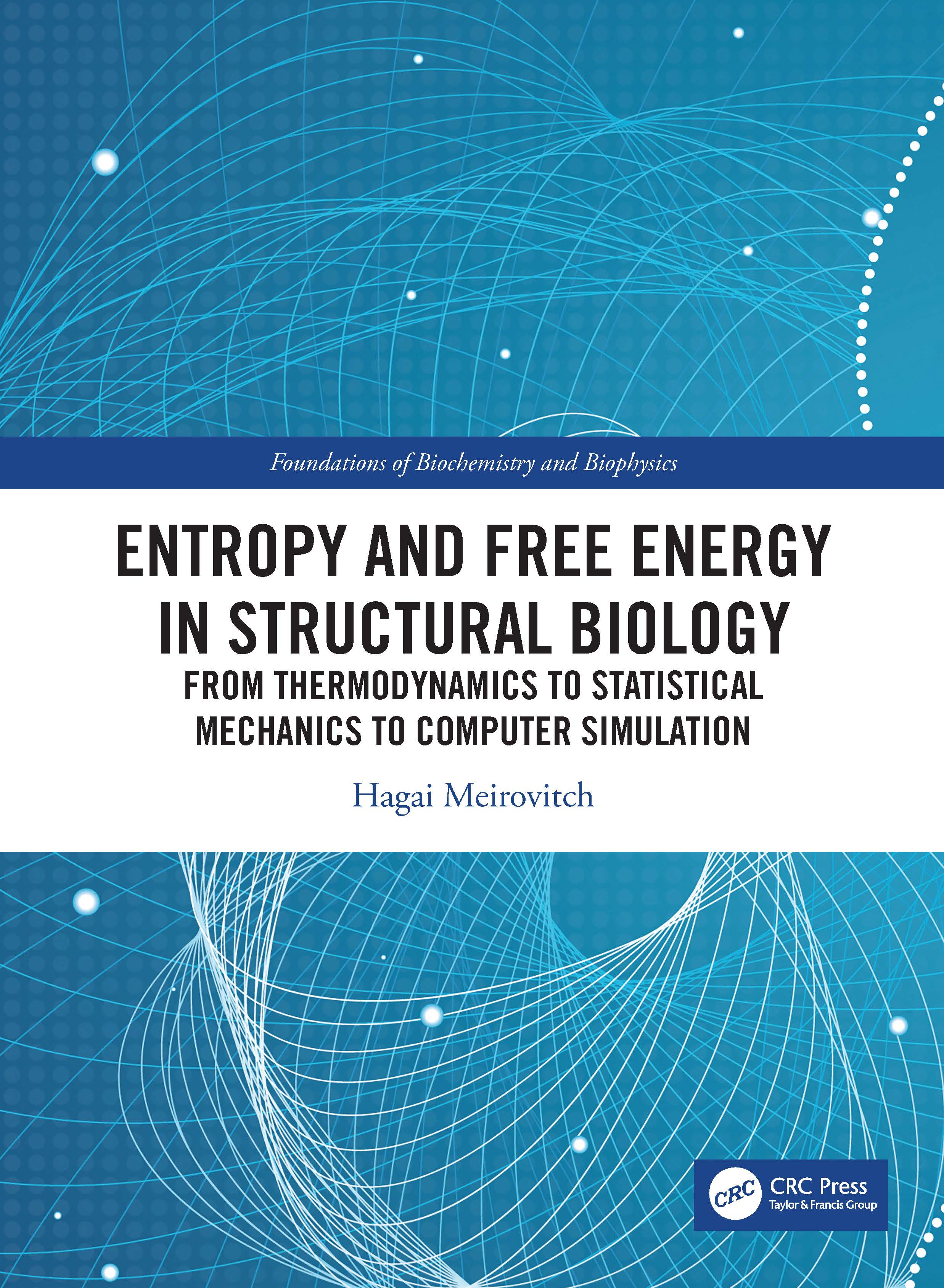# Entropy and Free Energy in Structural Biology

## From Thermodynamics, Statistical Mechanics and Computer Simulation, 1st Edition

CRC Press

288 pages

Hardback: 9780367406929
pub: 2020-06-16
Available for pre-order. Item will ship after 16th June 2020
\$130.00
x

FREE Standard Shipping!

### Description

Computer simulation has become the main engine of development in statistical mechanics. In structural biology, computer simulation constitutes the main theoretical tool for structure determination of proteins and for calculation of the free energy of binding, which are important in drug design. Entropy and Free Energy in Structural Biology leads the reader to the simulation technology in a systematic way. The book, which is structured as a course, consists of four parts:

Part I is a short course on probability theory emphasizing (1) the distinction between the notions of experimental probability, probability space, and the experimental probability on a computer, and (2) elaborating on the mathematical structure of product spaces. These concepts are essential for solving probability problems and devising simulation methods, in particular for calculating the entropy.

Part II starts with a short review of classical thermodynamics from which a non-traditional derivation of statistical mechanics is devised. Theoretical aspects of statistical mechanics are reviewed extensively.

Part III covers several topics in non-equilibrium thermodynamics and statistical mechanics close to equilibrium, such as Onsager relations, the two Fick's laws, and the Langevin and master equations. The Monte Carlo and molecular dynamics procedures are discussed as well.

Part IV presents advanced simulation methods for polymers and protein systems, including techniques for conformational search and for calculating the potential of mean force and the chemical potential. Thermodynamic integration, methods for calculating the absolute entropy, and methodologies for calculating the absolute free energy of binding are evaluated.

Enhanced by a number of solved problems and examples, this volume will be a valuable resource to advanced undergraduate and graduate students in chemistry, chemical engineering, biochemistry biophysics, pharmacology, and computational biology.

PART I. Probability Theory 1. Probability and its applications PART II. Equilibrium Thermodynamics and Statistical Mechanics 2. Classical Thermodynamics 3. From Thermodynamics to Statistical mechanics 4. Ideal gas and the harmonic Oscillator 5. Fluctuations and the most probable energy 6. Various ensembles 7. Phase transitions 8. Ideal polymer chains 9. Chains with excluded volume PART III. Basic Simulation Techniques and Non-Equilibrium Problems 10. The Metropolis Monte Carlo and molecular dynamics 11. Non-equilibrium thermodynamics- Onsager theory 12. Non-equilibrium statistical mechanics 13. The Master Equation PART IV. Advanced simulation methods. polymers and biological macromolecus 14. Growth simulation methods for polymers 15. The pivot algorithm and hybrid techniques 16. Models of proteins 17. Calculation of the entropy and the free energy by thermodynamic integration 18. Direct calculation of the absolute entropy and free energy 19. Calculation of the absolute entropy from a single Boltzmann sample 20. The potential of mean force, umbrella sampling and related techniques 21Advanced simulation and free energy techniques 22. Simulation of the chemical potential 23. The absolute free energy of binding

Hagai Meirovitch is Professor Emeritus in the Department of Computational and Systems Biology at the University of Pittsburgh.